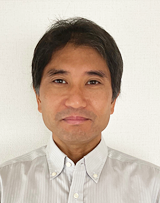Quantum state estimationGetting the most out of quantum systems

Quantitative information about a physical system comes in the form of numbers following from the system’s mathematical description — equations capturing the physical processes involved. In classical physics, it is in principle possible to retrieve, by means of measurements, the complete information of a system. According to quantum mechanics, however, one can never obtain all information of a system with infinite precision because of the quantum-mechanical uncertainty principle stating that certain pairs of quantities (e.g. momentum and position) cannot be measured simultaneously with absolute certainty.

Also, in quantum mechanics, measurements affect the state of a system, so it is actually impossible to determine a system’s state without prior knowledge about it. Quantum state estimation is the statistical framework addressing the question how to obtain the most — but, unavoidably, still incomplete — information about a quantum system from measurements.

Now, Jun Suzuki from the University of Electro-Communications and colleagues have extended the framework to include the situation of so-called ‘nuisance parameters’: parameters that are not really of interest but do affect the precision of estimations of other parameters that are of interest.

Nuisance parameters have been extensively studied in the context of classical physics and statistics. A key theoretical result is the Cramér-Rao inequality, providing a lower bound on the variance of the estimated value of a parameter. (The variance of a parameter is the expectation value of the square of the deviation from its mean value.) Suzuki and colleagues derived a scheme to obtain quantum Cramér-Rao bounds in the framework of quantum state estimation taking the presence of nuisance parameters into account.

After developing the general formalism, the researchers focused on the one-parameter case: the situation where only one parameter is of interest, but one or more nuisance parameters exist alongside. They succeeded in obtaining a straightforward ‘recipe’ for calculating the ultimate precision limit for the one parameter of interest. They applied their scheme for the description of a noisy quantum clock, as well as that of a so-called ‘qudit’, a multi-state generalization of a qubit. (The latter refers to a quantum-mechanical two-state system, like the spin of an electron, and is the fundamental concept underlying quantum computing.)

Suzuki and colleagues point out that their findings are relevant for quantum metrology. Quantum metrology essentially deals with estimating, with high sensitivity and high precision, parameters in quantum-mechanical physical processes by utilizing quantum resources. One of the main issues in this field is to perform quantum metrological tasks in a noisy environment; the noise thus depends on unknown nuisance parameters. Quoting the researchers: “Suitable extensions of the tools presented … will, therefore, be able to quantify the effects of nuisance parameters in quantum metrology.”

References